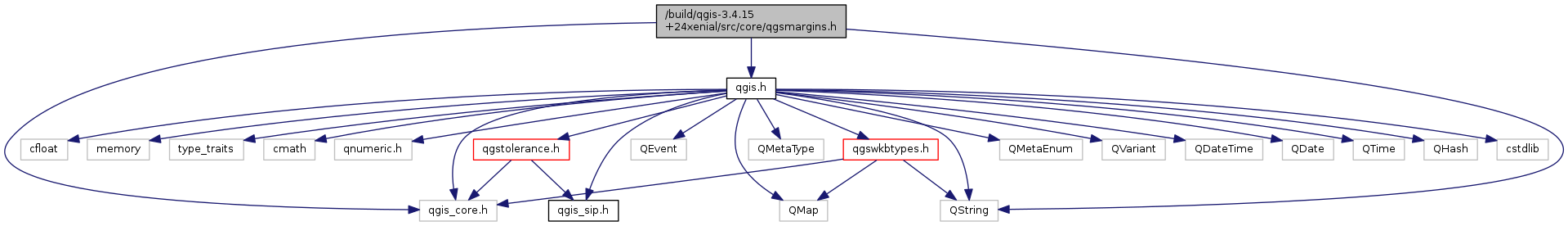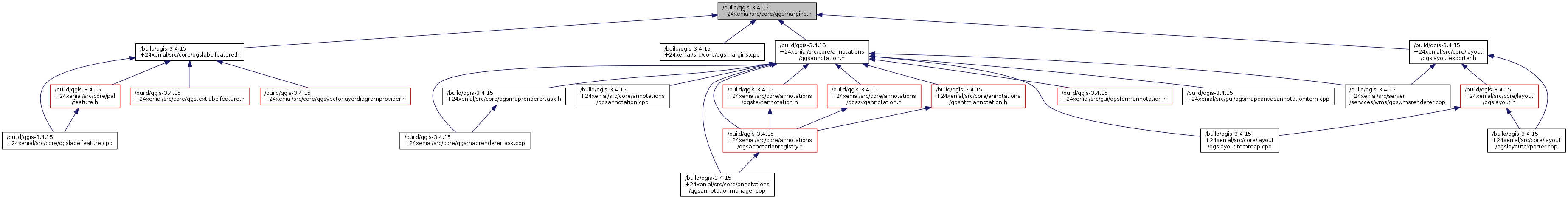qgsmargins.h File Reference
`#include "qgis_core.h"`
`#include "qgis.h"`
`#include <QString>`
Include dependency graph for qgsmargins.h:This graph shows which files directly or indirectly include this file:Go to the source code of this file.

## Classes

class  QgsMargins
The QgsMargins class defines the four margins of a rectangle. More...

## Functions

bool operator!= (const QgsMargins &lhs, const QgsMargins &rhs)
Returns `true` if lhs and rhs are different; otherwise returns `false`. More...

QgsMargins operator* (const QgsMargins &margins, double factor)
Returns a QgsMargins object that is formed by multiplying each component of the given margins by factor. More...

QgsMargins operator* (double factor, const QgsMargins &margins)
Returns a QgsMargins object that is formed by multiplying each component of the given margins by factor. More...

QgsMargins operator+ (const QgsMargins &m1, const QgsMargins &m2)
Returns a QgsMargins object that is the sum of the given margins, m1 and m2; each component is added separately. More...

QgsMargins operator+ (const QgsMargins &lhs, double rhs)
Returns a QgsMargins object that is formed by adding rhs to lhs. More...

QgsMargins operator+ (double lhs, const QgsMargins &rhs)
Returns a QgsMargins object that is formed by adding lhs to rhs. More...

QgsMargins operator+ (const QgsMargins &margins)
Returns a QgsMargins object that is formed from all components of margins. More...

QgsMargins operator- (const QgsMargins &m1, const QgsMargins &m2)
Returns a QgsMargins object that is formed by subtracting m2 from m1; each component is subtracted separately. More...

QgsMargins operator- (const QgsMargins &lhs, double rhs)
Returns a QgsMargins object that is formed by subtracting rhs from lhs. More...

QgsMargins operator- (const QgsMargins &margins)
Returns a QgsMargins object that is formed by negating all components of margins. More...

QgsMargins operator/ (const QgsMargins &margins, double divisor)
Returns a QgsMargins object that is formed by dividing the components of the given margins by the given divisor. More...

bool operator== (const QgsMargins &lhs, const QgsMargins &rhs)
Returns `true` if lhs and rhs are equal; otherwise returns `false`. More...

Q_DECLARE_TYPEINFO (QgsMargins, Q_MOVABLE_TYPE)

## Function Documentation

 bool operator!= ( const QgsMargins & lhs, const QgsMargins & rhs )
inline

Returns `true` if lhs and rhs are different; otherwise returns `false`.

Definition at line 186 of file qgsmargins.h.

 QgsMargins operator* ( const QgsMargins & margins, double factor )
inline

Returns a QgsMargins object that is formed by multiplying each component of the given margins by factor.

Definition at line 242 of file qgsmargins.h.

 QgsMargins operator* ( double factor, const QgsMargins & margins )
inline

Returns a QgsMargins object that is formed by multiplying each component of the given margins by factor.

Definition at line 252 of file qgsmargins.h.

 QgsMargins operator+ ( const QgsMargins & m1, const QgsMargins & m2 )
inline

Returns a QgsMargins object that is the sum of the given margins, m1 and m2; each component is added separately.

Definition at line 195 of file qgsmargins.h.

 QgsMargins operator+ ( const QgsMargins & lhs, double rhs )
inline

Returns a QgsMargins object that is formed by adding rhs to lhs.

Definition at line 214 of file qgsmargins.h.

 QgsMargins operator+ ( double lhs, const QgsMargins & rhs )
inline

Returns a QgsMargins object that is formed by adding lhs to rhs.

Definition at line 223 of file qgsmargins.h.

 QgsMargins operator+ ( const QgsMargins & margins )
inline

Returns a QgsMargins object that is formed from all components of margins.

Definition at line 309 of file qgsmargins.h.

 QgsMargins operator- ( const QgsMargins & m1, const QgsMargins & m2 )
inline

Returns a QgsMargins object that is formed by subtracting m2 from m1; each component is subtracted separately.

Definition at line 205 of file qgsmargins.h.

 QgsMargins operator- ( const QgsMargins & lhs, double rhs )
inline

Returns a QgsMargins object that is formed by subtracting rhs from lhs.

Definition at line 232 of file qgsmargins.h.

 QgsMargins operator- ( const QgsMargins & margins )
inline

Returns a QgsMargins object that is formed by negating all components of margins.

Definition at line 317 of file qgsmargins.h.

 QgsMargins operator/ ( const QgsMargins & margins, double divisor )
inline

Returns a QgsMargins object that is formed by dividing the components of the given margins by the given divisor.

Definition at line 262 of file qgsmargins.h.

 bool operator== ( const QgsMargins & lhs, const QgsMargins & rhs )
inline

Returns `true` if lhs and rhs are equal; otherwise returns `false`.

Definition at line 175 of file qgsmargins.h.

 Q_DECLARE_TYPEINFO ( QgsMargins , Q_MOVABLE_TYPE )